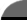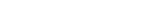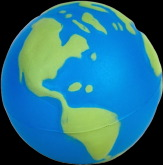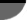Facts By Category:» Physics  » Astronomy  » Chemistry  » Biology  » Mathematics  » Geology  » Engineering  » Medicine  » Science   by keyword search:       ScienceIQ Team:»Writers & EditorsxUmp.com
Science Supplies,

Physics & Astronomy
Online
How To Calculate The Area Of A CircleA circle is the round counterpart of a square. To find the area of a square, one multiplies the length by the width. A circle doesn't have these, however, so there has to be a different way to calculate the area. To visualize how the area of a circle is derived, think about how a circle can be made. A circle has a center point, and every point on the edge of the circle is exactly the same distance from this central point. Now imagine a straight line that extends from the center point to the edge of the circle. This is called the radius. Now imagine that line swinging all the way around one end until it comes right back to where it started. As it swings around it paints the area that it has gone over. This describes a circle, but not just the outside edge of the circle. This describes all the area contained within the circle as well.

As the line moves around, a point is reached where the area that has been painted by the line is equal to the square value of the radius. Here the color of the paint changes. As the line continues to sweep around, another such segment gets painted, and then another. At this point the area that is left to paint before the circle is finished is much smaller than each square radius segment. The number of these segments in the area of the circle turns out to be equal to p. As this 'sweeping line' description demonstrates, the area of a circle is equal to the sum of p segments, each of which has an area equal to the square of the radius. The area of a circle is then given by the general equation A = pr2.

As an example of how to use this equation, imagine that you have a circular flower bed that is 20 feet in diameter, and you need to apply a layer of enriched soil. One bag contains enough of the soil to cover 10 square feet to the thickness you want, so how many bags of soil will you need? (Use p = 3.14). The radius of the flower bed is half of the diameter, or 10 feet. Substituting these values into the formula shows the area of the flower bed to be A = p X r X r. So A equals 3.14 X 10 X 10, which equals 314 square feet. You will therefore have to buy 31.4 bags of enriched soil (you could get 32 and spread the extra a little thicker, or 31 and not use quite so much...).

474Richard M. J. Renneboog, MS Richard M. J. Renneboog is an independent private technical consultant and writer in both chemical and computer applications. Endeavors have included preparation of scripts for instructional and promotional video, corporate website design, curriculum development for training in advanced composites technology, and development.The Joy of Piby David Blatner
 Home | Privacy Policy | Cookie PolicyCopyright © 2002-2019 ScienceIQ.com - All Rights Reserved